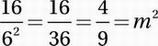# SAT Math Multiple Choice Question 675: Answer and Explanation

### Test Information

Question: 675

15. If (6-2)(m-2) =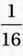, what is the value of m2?

• A.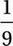• B.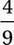• C.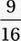• D.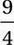Explanation:

B

For this one, we'll need the Laws of Exponentials from

Chapter 9, Lesson 9.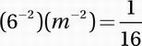Translate by using Exponential Law #3: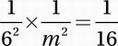Multiply by m2: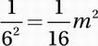Multiply by 16: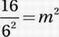Simplify: